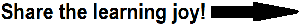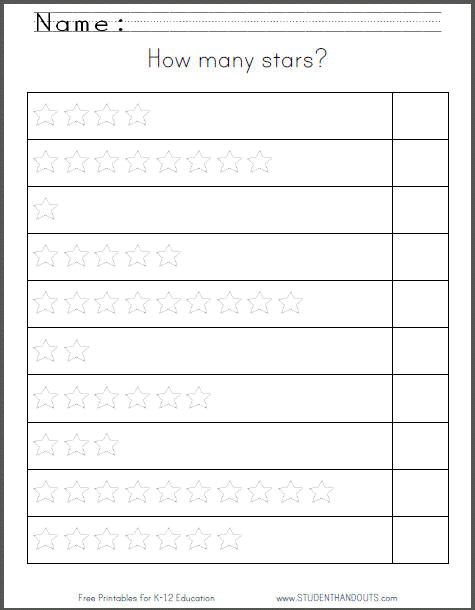﻿
How many stars? Printable Counting Worksheet | Student HandoutsHow many stars? Counting WorksheetThis free printable worksheet has kids count stars. Each row displays a number of stars between one and ten. In the box to the right, the student writes down the corresponding number. Click here to print. Click here for more of our free kindergarten counting worksheets.
 CCSS.MATH.CONTENT.K.CC.A.3 - Write numbers from 0 to 20. Represent a number of objects with a written numeral 0-20 (with 0 representing a count of no objects).
 CCSS.MATH.CONTENT.K.CC.B.5 - Count to answer "how many?" questions about as many as 20 things arranged in a line, a rectangular array, or a circle, or as many as 10 things in a scattered configuration; given a number from 1-20, count out that many objects.